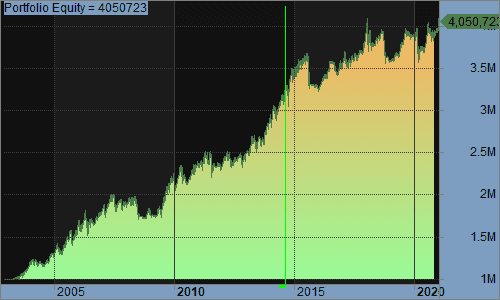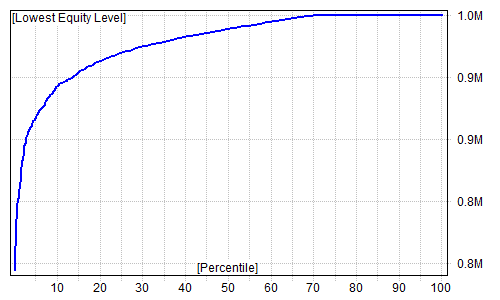# version 45 economic notes

I revised the economic indicators for accuracy. The RAR increased to 9.82% with 82% exposure. MDD is -16.23%, relatively unchanged. Two open Bond positions with BNDX and MUB.

Economic Interpretation is 6-3 in favor of indicating that we are in an expansion period, as follows:

Version45
Phase 1: Expansion leaving Trough
Phase 2: Expansion approaching Peak
Phase 3: Contraction leaving Peak
Phase 4: Contraction approaching Trough

DGS3MO 90-day Treasury Indicator
DGS3MOprice=0.1
Phase Prediction=1

DBC COMMODITY INDICATOR
DBC price=15.3
Phase Prediction=2

SPY price=375.7
Phase Prediction=2

SPY price=6.7
Phase Prediction=2

HOUST HOUSING STARTS CONFIRMATION
HOUST price=1547
Phase Prediction=3

VIXCLS CBOE Volatility Indicator
Phase Prediction=3

CP CORPORATE PROFITS CONFIRMATION
CP price=2118.86
Phase Prediction=3

TWEXM US DOLLAR INDICATOR
TWEXM price=91.5077
Phase Prediction=2

XLU UTILITIES INDICATED PHASE
XLU price=62.99
Phase Prediction=1

Phase Predictor Indicator
Phase 1 Total = 2
Phase 2 Total = 4
Phase 3 Total = 3
Phase 4 Total = 0

Expansion Ind = 1 Contraction Ind = 0